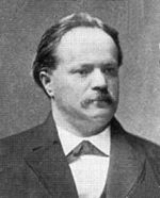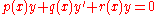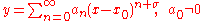xLazarus FuchsEncyclopedia
Lazarus Immanuel Fuchs was a German
German language
German is a West Germanic language, related to and classified alongside English and Dutch. With an estimated 90 – 98 million native speakers, German is one of the world's major languages and is the most widely-spoken first language in the European Union....

mathematician
Mathematician
A mathematician is a person whose primary area of study is the field of mathematics. Mathematicians are concerned with quantity, structure, space, and change....

who contributed important research in the field of linear differential equation
Linear differential equation
Linear differential equations are of the formwhere the differential operator L is a linear operator, y is the unknown function , and the right hand side ƒ is a given function of the same nature as y...

s. He was born in Mosina
Mosina
Mosina is a town in Poznań County, Greater Poland Voivodeship, Poland, about 20 km south Poznań, with 12,107 inhabitants .-External links:*...

(located in Grand Duchy of Poznań
Grand Duchy of Poznan
The Grand Duchy of Posen, or the Grand Duchy of Poznań was part of the Kingdom of Prussia, created from territories annexed by Prussia after the Partitions of Poland, and formally established following the Napoleonic Wars in 1815. Per agreements derived at the Congress of Vienna it was to have...

) and died in Berlin
Berlin
Berlin is the capital city of Germany and is one of the 16 states of Germany. With a population of 3.45 million people, Berlin is Germany's largest city. It is the second most populous city proper and the seventh most populous urban area in the European Union...

, Germany.

He is the eponym of Fuchsian group
Fuchsian group
In mathematics, a Fuchsian group is a discrete subgroup of PSL. The group PSL can be regarded as a group of isometries of the hyperbolic plane, or conformal transformations of the unit disc, or conformal transformations of the upper half plane, so a Fuchsian group can be regarded as a group acting...

s and functions, and the Picard–Fuchs equation; Fuchsian differential equation
Differential equation
A differential equation is a mathematical equation for an unknown function of one or several variables that relates the values of the function itself and its derivatives of various orders...

s are those with regular singularities. Fuchs is also known for Fuchs's theorem
Fuchs's theorem
In mathematics, the Fuchs' theorem, named after Lazarus Fuchs, states that a second order differential equation of the formy + py'+qy=g\;...

which states that if x0 is a regular singular point then the differential equationhas at least one solution of the formfor some σ to be determined. In some cases, there will be two linearly independent
Linear independence
In linear algebra, a family of vectors is linearly independent if none of them can be written as a linear combination of finitely many other vectors in the collection. A family of vectors which is not linearly independent is called linearly dependent...

solutions of that form.

## Selected works

• Über Funktionen zweier Variabeln, welche durch Umkehrung der Integrale zweier gegebener Funktionen entstehen, Göttingen 1881.
• Zur Theorie der linearen Differentialgleichungen, Berlin 1901.
• Gesammelte Werke, Hrsg. von Richard Fuchs und Ludwig Schlesinger. 3 Bde. Berlin 1904–1909.

## External links

The source of this article is wikipedia, the free encyclopedia.  The text of this article is licensed under the GFDL.